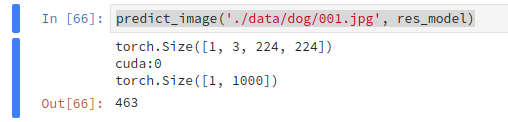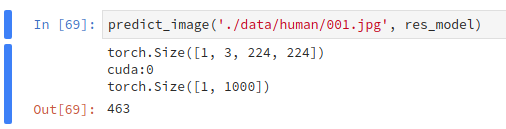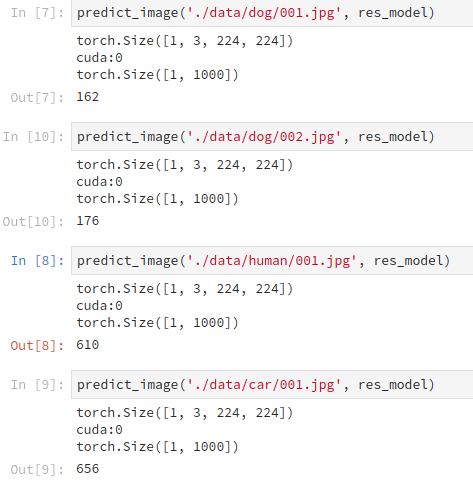# Get same output with different image on pre-trained ResNet18

I used `torchvision.model.resnet18` to classify images, but it seems output same result with different input.
Here is my test code

``````res_model = torchvision.models.resnet18(pretrained=True)
normalize = transforms.Compose([
transforms.Resize((224, 224)),
transforms.ToTensor(),
transforms.Normalize(
mean=[0.485, 0.456, 0.406],
std=[0.229, 0.224, 0.225]
)
])
res_model.to(device)
def predict_image(image_path, model):
image = Image.open(image_path)
image_tensor = normalize(image).float()
image_tensor = image_tensor.unsqueeze_(0)
image_tensor = image_tensor.to(device)
output = model(image_tensor)
output_c = output.cpu()
index = output_c.data.numpy().argmax()
return index

``````

Here are some test case with different images and the same outputThough I don’t know what the index means, I think the same index means the same category.

Did I do something wrong?

Try to set your model to evaluation using `model.eval()` before testing your images.